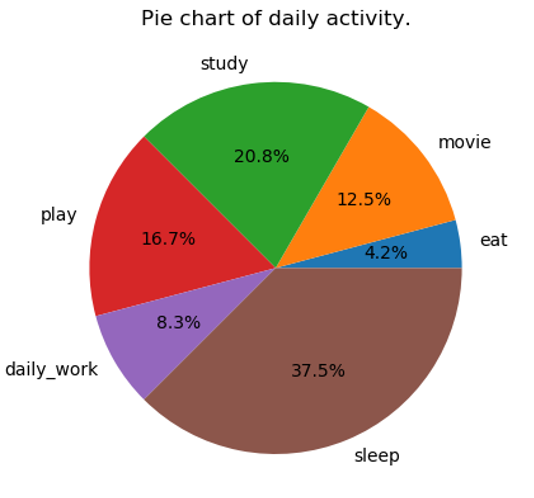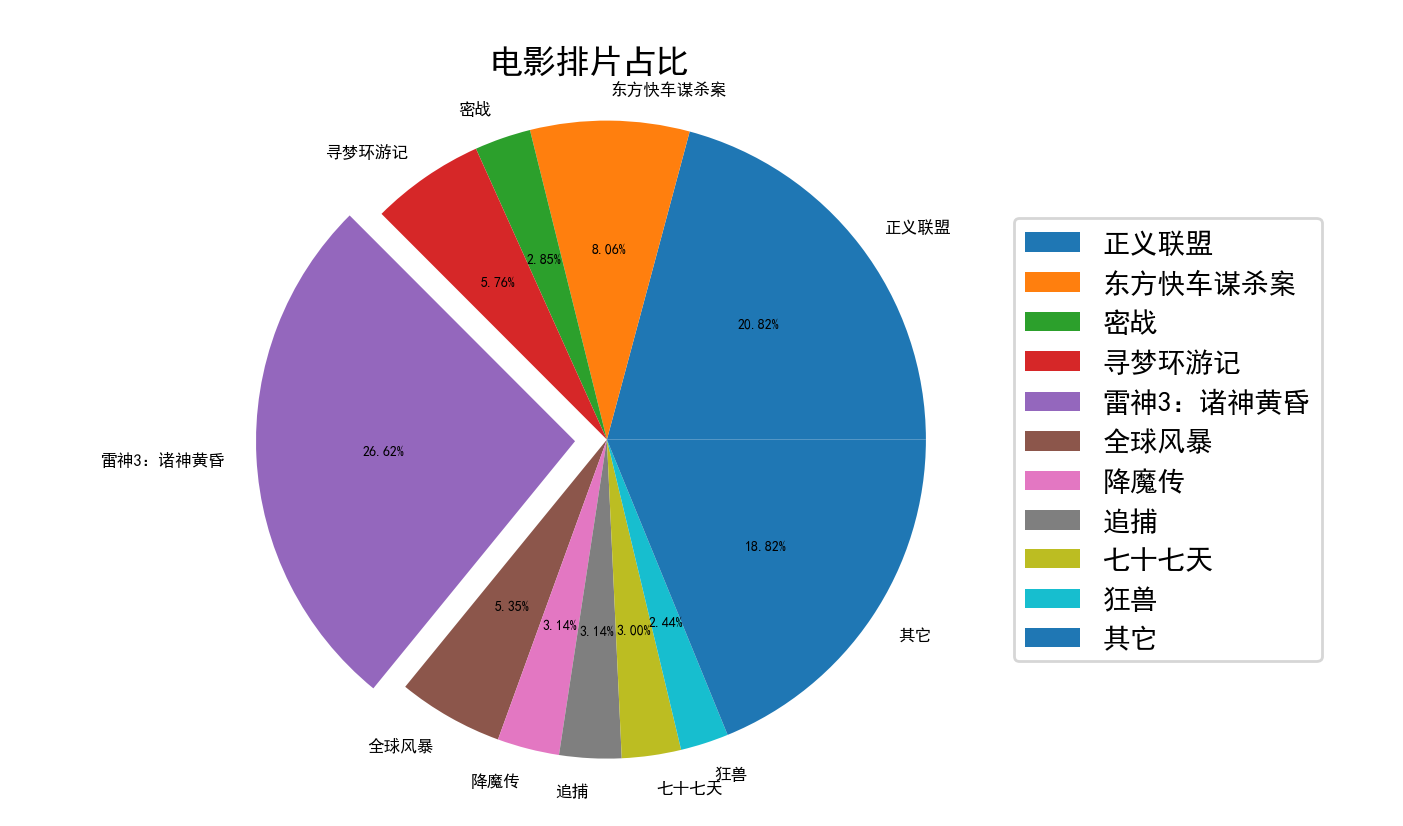• python绘制饼图 如果您是视觉学习者，这里是嵌入式视频 饼形图： 饼图是圆形统计图。 整个图表的区域代表100％或全部数据。 饼图中显示的饼图区域代表数据部分的百分比。 饼图的各个部分称为楔形。 ...

python绘制饼图

如果您是视觉学习者，这里是嵌入式视频

饼形图：

• 饼图是圆形统计图。
• 整个图表的区域代表100％或全部数据。
• 饼图中显示的饼图区域代表数据部分的百分比。
• 饼图的各个部分称为楔形。
• 楔形的弧长决定饼图中楔形的面积。
• 楔形的面积决定了零件相对于整体的相对量子或百分比。
• 饼图经常用于业务演示中，因为它们可以快速概述业务活动，例如销售，运营等。
• 饼形图还大量用于调查结果，新闻文章，资源使用图（如磁盘和内存）中。

使用Python Matplotlib绘制简单的饼图

可以使用pyplot模块中的函数pie（）绘制饼图。 以下python代码示例使用pie（）函数绘制了一个饼图。

默认情况下，pyplot的pie（）功能沿逆时针方向排列饼图中的饼形或楔形。

例：

# import the pyplot library
import matplotlib.pyplot as plotter
# The slice names of a population distribution pie chart
pieLabels              = 'Asia' , 'Africa' , 'Europe' , 'North America' , 'South America' , 'Australia'
# Population data
populationShare     = [ 59.69 , 16 , 9.94 , 7.79 , 5.68 , 0.54 ]
figureObject, axesObject = plotter.subplots()
# Draw the pie chart
axesObject.pie(populationShare,
labels=pieLabels,
autopct= '%1.2f' ,
startangle= 90 )
# Aspect ratio - equal means pie is a circle
axesObject.axis( 'equal' )
plotter.show()

输出：在饼图中创建楔形以爆炸：

使用饼图函数的explode参数，可以使饼图的楔形从饼图的其余楔形中爆炸。

explode是一个元组，其中每个元素都对应于饼图的楔形。

元组的长度应等于饼图中的饼图数。

否则， matplotlib将引发ValueError类型的异常。

例：

explodeTuple = (0.1 , 0.0 , 0.0 , 0.0 , 0.0 , 0.0 )
# Draw the pie chart
axesObject.pie(populationShare, explode=explodeTuple,
labels=pieLabels,
autopct= '%1.2f' ,
startangle= 90 )

在上面的代码片段中，pie（）函数与元组一起传递给explode参数。 元组中只有第一个元素是小数，而其他所有元素都为零，这只会使饼图的第一个楔形与图表的其余部分分开。

输出：使饼图的多个楔形爆炸：

上面的示例仅使饼图的楔形之一爆炸。

在以下代码段中，为pie（）函数的explode参数提供了一个元组。 元组的第一个和最后一个条目为非零分数，从而使饼图的第一个和最后一个楔形爆炸。

基于元组中元素的数值，楔形会爆炸到离饼图中心点相对较高或较低的距离。

负值将使楔形出现在与指定的原始位置相反的方向上。

例：

explodeTuple = (0.1 , 0.0 , 0.0 , 0.0 , 0.0 , 0.8 )
# Draw the pie chart
axesObject.pie(populationShare, explode=explodeTuple,
labels=pieLabels,
autopct= '%1.2f' ,
startangle= 90 )

输出：在使用Matplotlib绘制的饼图中自定义颜色：

可以使用pie（）函数的参数颜色自定义饼图中的楔形颜色。 colors参数是一个元组，其中包含要为饼图的楔形循环的颜色列表。

例：

# import the pyplot library
import matplotlib.pyplot as plotter
# Guest age group
ageGroupLabel = 'Below 5' , '5-10' , '10-15' , '15-20' , '20-30' , '30-40' , '40-50' , '50-60' , '60-80' , '80-100' , 'Above 100'
# Number of Guests expected in age group
guestNumbers     = [ 5 , 10 , 10 , 15 , 10 , 30 , 25 , 25 , 20 , 15 , 10 ]
figureObject, axesObject = plotter.subplots()
explode = ( 0.4 , 0.0 , 0.0 , 0.0 , 0.5 , 0.5 , 0.0 , 0.0 , 0.0 , 0.0 , 0.3 )
colors  = ( "red" , "green" , "orange" , "cyan" , "brown" , "grey" , "blue" , "indigo" , "beige" , "yellow" )
# Draw the pie chart
axesObject.pie(guestNumbers,
explode      = explode,
colors       = colors,
labels       = ageGroupLabel,
autopct      = '%1.2f' ,
startangle   = 90 )
# Aspect ratio
axesObject.axis( 'equal' )
plotter.show()

输出：在输出中，由于在达到“ 100岁以上”年龄组时所有颜色均已用尽，因此将从头开始再次进行颜色设置，即将红色分配给与该年龄段的孩子相同的“ 100岁以上”年龄组。

自定义Matplotlib饼图：

• 可以自定义Matplotlib.pyplot绘制的饼图的几个方面。
• startangle参数将饼图旋转指定的度数。 旋转是逆时针方向，并在饼图的X轴上执行。
• 可以使用楔子属性参数进一步定制饼图的楔子。 可以使用名称值对描述楔形属性（例如edgecolor，linewidth）的python字典作为楔形属性参数来传递。
• 通过将frame参数设置为True，将围绕饼图绘制轴框架。
• arc（）函数的Autopct参数控制百分比在楔形中的显示方式。 可以指定以％开头的格式字符串，也可以指定函数。 例如，％。1f将以25.0、35.2等格式显示百分比值。 ％.2f %%将以50.25、75.5等格式显示百分比值。

例：

import matplotlib.pyplot as plotter
# Distribution of n-grams in a paragraph - maximum wordlength 5
wedgeLabels     = ( "Unigram" , "Bigram" , "Trigram" , "Four-gram" , "Five-gram" , "Others" )
# Percentage of n-grams
ngramPercent    = ( 5 , 5 , 10 , 5 , 10 , 65 )
figureObject, axesObject = plotter.subplots()
axesObject.pie(ngramPercent,
labels       = wedgeLabels,
frame        = True ,
startangle   = 120 ,
autopct      = '%.1f%%' ,
wedgeprops   = { 'linewidth' : 3 ,
'edgecolor' : "orange" })
axesObject.axis( 'equal' )
plotter.show()

输出：python绘制饼图

展开全文python java mybatis canvas django
• 原标题：Python入门进阶：Python绘制饼图到Microsoft Excel来自：Linux迷https://www.linuxmi.com/python-pie-chart-microsoft-excel.html在本文中，我想向您展示使用Python向Microsoft Excel绘制饼图，为此我们将...

原标题：Python入门进阶：Python绘制饼图到Microsoft Excel

来自：Linux迷https://www.linuxmi.com/python-pie-chart-microsoft-excel.html

在本文中，我想向您展示使用Python向Microsoft Excel绘制饼图，为此我们将使用XlsxWriter库。XlsxWriter是一个Python模块，用于编写Excel 2007+ XLSX文件格式的文件。XlsxWriter可以用来编写文本，数字，公式和超链接到多个工作表，它支持的功能，如格式和更多，包括:

100%兼容Excel XLSX文件。

完整的格式。

合并单元格。

定义的名字。

图表。

自动筛选。

数据验证和下拉列表。

条件格式。

工作表PNG和JPEG/BMP/WMF/EMF图像。

丰富的多格式字符串。

单元格注释。

与Pandas集成。

文本框。

支持添加宏。

用于写入大文件的内存优化模式。

它支持Python 2.7、3.4+和PyPy，并且只使用标准库。

首先，您需要安装这个库

pip install XlsxWriter

下面是本文的完整代码

# 导入xlsxwriter模块

importxlsxwriter

# Workbook 接受一个非可选参数

# 这是我们要创建的文件名。

workbook = xlsxwriter.Workbook( "www.linuxmi.com.xlsx")

# 然后使用工作簿对象添加新的

# 这里我们创建粗体格式对象。

# 这是我们的数据和数据列表

data = [

[ 'LinuxMi', 'Ubunut', 'Python'],

[ 55, 35, 6],

]

# 从“A1”开始写入一行数据

# 粗体格式。

# 从开始写入一列数据

# 分别为A2、B2、C2。

worksheet.write_column( 'A2', data[ 0])

worksheet.write_column( 'B2', data[ 1])

# 这是图表类型

# 向图表添加数据系列

'name': 'Pie Sales Data',

'categories':[ 'Sheet1', 1, 0, 3, 0],

'values':[ 'Sheet1', 1, 1, 3, 1],

})

# 设置图表的标题

chart1.set_title({ 'name': 'www.linuxmi.com - Data Chart'})

# 设置图表的样式

chart1.set_style( 10)

# 将图表插入工作表

worksheet.insert_chart( 'C2', chart1, { 'x_offset': 25, 'y_offset': 10})

# 关闭工作簿

workbook.close

运行完整的代码，结果如下：责任编辑：

展开全文• python绘制饼图The pie chart represents the quantity in percentages, and the total sum of all the segments of the pie chart must be equal to 100%. It is used to visualize the given data in percentage. ...

python绘制饼图

The pie chart represents the quantity in percentages, and the total sum of all the segments of the pie chart must be equal to 100%. It is used to visualize the given data in percentage. The user will provide us one list of daily activities and another list that shows the time taken daily to do each activity and by using these lists we have to draw a pie chart by using the Python. One thing will come to your mind that to draw a pie chart of daily activity, initially, we have to calculate the percentage of each activity time then draw the pie chart and it will take a lot of time. Yes, this approach definitely will take a lot of time and that's why we will not go for this approach. So, don't worry about it because Python provides us an in-built matplotlib library which makes it so much easier. Before using the matplotlib library in the program, we will see a little bit about it and the installation process.

饼图以百分比表示数量，并且饼图所有段的总和必须等于100％。 它用于以百分比显示给定的数据。 用户将向我们提供一个日常活动列表，以及另一个列表，该列表显示了每天执行每个活动所花费的时间，通过使用这些列表，我们必须使用Python绘制饼图。 您会想到一件事，要绘制每日活动的饼图，首先，我们必须计算每个活动时间的百分比，然后绘制饼图，这将花费大量时间。 是的，这种方法肯定会花费很多时间，这就是为什么我们不会采用这种方法的原因。 因此，不必担心，因为Python为我们提供了内置的matplotlib库 ，这使它变得更加容易。 在程序中使用matplotlib库之前，我们将了解一些有关它和安装过程的信息。

matplotlib library is one of the most useful libraries of Python. It is used for visualization of given data in 2D plots. By using this, we can draw plots, pie charts, histograms, scatterplots, etc.

matplotlib库是Python最有用的库之一。 它用于在2D图中可视化给定数据。 通过使用它，我们可以绘制图，饼图，直方图，散点图等。

Algorithm to solve this problem

解决这个问题的算法

1. Import pyplot module from the matplotlib library as plt.

matplotlib库 中将pyplot模块导入为plt

2. Take the daily activity and time taken to do the activity in two arrays.

以两个阵列为单位进行日常活动和花费的时间。

3. Now, draw the pie chart with the title 'Pie chart of daily activity'.

现在， 绘制标题为“每日活动饼图”的饼图

So, let's start writing a few lines of code in Python by implementing the above algorithm in a simple way.

因此，让我们以一种简单的方式实现上述算法，开始用Python写几行代码。

Program:

程序：

import matplotlib.pyplot as plt

A=['eat', 'movie', 'study', 'play','daily_work','sleep']
T=[1,3,5,4,2,9]

plt.pie(T, labels=A,autopct= '%1.1f%%')
plt.title('Pie chart of daily activity.')
plt.show()

Output

输出量python绘制饼图

展开全文算法 可视化 python java 机器学习
• 设置字体的大小patches,l_text,p_text=plt.pie(money_rate,explode=explode,labels=names,autopct='%.2f%%')# l_text是饼图对着文字大小，p_text是饼图内文字大小for t in p_text:t.set_size(5)for t in l_text:t....

设置字体的大小

patches,l_text,p_text=plt.pie(money_rate,explode=explode,labels=names,autopct='%.2f%%')

# l_text是饼图对着文字大小，p_text是饼图内文字大小

for t in p_text:

t.set_size(5)

for t in l_text:

t.set_size(6)

防止标签重叠，可以将窗口设置的大一些

plt.figure(figsize=(20, 6.5))

3.源码

import matplotlib

import matplotlib.pyplot as plt

import numpy as np

matplotlib.rcParams['font.family']='SimHei'

names=np.array(['正义联盟','东方快车谋杀案','密战','寻梦环游记','雷神3：诸神黄昏', '全球风暴', '降魔传','追捕','七十七天','狂兽','其它'])

money=np.array([57767,22354,7916,15969,73853,14839,8725,8716,8318,6764,52222])

money_rate=money/np.sum(money)

explode=np.zeros((len(money)))

explode=0.1

plt.figure(figsize=(20, 6.5))

patches,l_text,p_text=plt.pie(money_rate,explode=explode,labels=names,autopct='%.2f%%')

plt.legend(["雷神3：诸神黄昏","正义联盟","东方快车谋杀案","寻梦环游记","全球风暴","降魔传","追捕","七十七天","密战","狂兽","其它"])

plt.legend(loc='center right')

plt.title('电影排片占比')

plt.axis('equal')

# 设置饼图内文字大小

for t in p_text:

t.set_size(5)

for t in l_text:

t.set_size(6)

plt.show()

4.结果展开全文• values = [3, 12, 5, 8] labels = ['a', 'b', 'c', 'd'] def make_autopct(values): def my_autopct(pct): total = sum(values) val = int(round(pct*total/100.0)) # 同时显示数值和占比的饼图 return '{p:.2f}% ({...python 数据分析 可视化 数据可视化
• Python matplotlib绘制饼图

千次阅读 2020-11-14 01:06:35
• startangle=180,wedgeprops=dict(width=0.3,edgecolor="w"),autopct='%1.1f%%') 相关免费学习推荐：python视频教程 以上就是python使用Matplotlib绘制饼图的详细内容，更多请关注龙方网络其它相关文章！
• 1.饼图函数基本绘制import matplotlib.pyplot as pltshuju = [10,20,30,40] ----->编辑饼图的各个变量占比大小plt.pie(bili)plt.show()2.参数：explodeimport matplotlib.pyplot as pltshuju= [10,20,30,40]A = [0,...
• 本期，我们将详细学习matplotlib 绘制饼图相关属性的学习，let's go~ 1. 等高线图概述 什么是饼图？ 饼图将各项的大小与总项和的比例显示在一个圆里 饼图展示通过不同大小，来确定各项的占比 饼图相.python 开发语言 后端
• 介绍matplotlib 是python最著名的绘图库，它提供了一整套和matlab相似的命令API，十分适合交互式地进行制图。而且也可以方便地将它作为绘图控件，嵌入GUI应用程序中。它的文档相当完备，并且 Gallery页面 中有上百幅...
• 今天我们来学习一下如何使用Python的Plotly绘图工具，绘制饼图使用Plotly绘制饼图的方法，我们需要使用graph_objs中的Pie函数函数中最常用的两个属性values，用于赋值给需要可视化的数据另外一个属性labels则是表示...
• import matplotlib.pyplot as plt import matplotlib as mpl ...plt.figure(figsize=(6,6))#将画布设定为正方形，则绘制饼图是正圆 label=['篮球','羽毛球','足球']#定义饼图的名称 explode=[0.01,0.01,0..python
• maconomy2python 更新：“新”技术导出xlsx格式（在plotprojectdata.py中将use_csv = True设置为读取“旧” csv文件） 用法示例示例：python plotprojectdata.py --filename ExportProjectCard_projectnumber.xlsx...Pythonpython 后端
• 今天小编就为大家分享一篇关于Python饼状图的绘制实例，小编觉得内容挺不错的，现在分享给大家，具有很好的参考价值，需要的朋友一起跟随小编来看看吧
• 您应该查看domain参数来从piecharts生成子图。例如，要在一行(xaxis)中制作两个piechart，可以指定第一个和第二个绘图将占用多少位置(第一个从0%到50%，第二个从50%到100%)。在代码：from plotly import toolsimport...
• 今天我们来学习一下如何使用Python的Plotly绘图工具，绘制饼图使用Plotly绘制饼图的方法，我们需要使用graph_objs中的Pie函数函数中最常用的两个属性values，用于赋值给需要可视化的数据另外一个属性labels则是表示...
• 饼图（Pie Graph）用于显示一个数据系列中各项的大小及其在各项总和中所占的比例。...pyplot 中绘制饼图的函数为 pie，其语法格式如下：matplotlib.pyplot.pie(x,explode=None,labels=None,colo......

python绘制饼图python 订阅